# Difference between revisions of "Paracompact Hausdorff implies normal"

This article gives the statement and possibly, proof, of an implication relation between two topological space properties. That is, it states that every topological space satisfying the first topological space property (i.e., paracompact Hausdorff space) must also satisfy the second topological space property (i.e., normal space)
View all topological space property implications | View all topological space property non-implications
Get more facts about paracompact Hausdorff space|Get more facts about normal space

## Statement

Any paracompact Hausdorff space (i.e., a space that is both paracompact and Hausdorff) is a normal space.

## Proof

Given: A paracompact Hausdorff space$X$.

To prove:$X$ is a normal space.

Proof: We first prove that$X$ is a regular space, and then prove that$X$ is normal.

### Proof of regularity

To prove: If$x \in X$ and$A$ is a closed set not containing$x$, there exist open subsets$U,V \subseteq X$ such that$x \in U, A \subseteq V$, and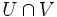$U \cap V$ is empty.

Proof:

1. By Hausdorffness, we can define, for every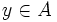$y \in A$, open subsets$U_y, V_y$ such that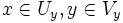$x \in U_y, y \in V_y$, and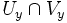$U_y \cap V_y$ is empty.
2. The open subsets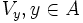$V_y, y \in A$, cover$A$. In other words,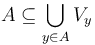$A \subseteq \bigcup_{y \in A} V_y$.
3. The sets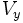$V_y$ and$X \setminus A$ form an open cover of$X$. Thus, by paracompactness of$X$, there is a locally finite open refinement. Throwing out from this any open subset not intersecting$A$, we still get a locally finite collection$\mathcal{P}$ of open subsets, each contained in some$V_y$, that cover$A$.
4. There exists an open set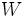$W$ containing$x$ such that there are only finitely many members of$\mathcal{P}$ that intersect$W$: This follows from the definition of local finiteness.
5. Let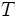$T$ be a finite subset of$A$ that contains, for each of this finite list of members of$\mathcal{P}$, a point$y$ such that that member is contained in$V_y$.
6. Define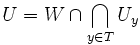$U = W \cap \bigcap_{y \in T} U_y$ and$V$ to be the union of all the members of$\mathcal{P}$. Then,$x \in U, A \subseteq V$, and$U$ and$V$ are disjoint: For this, note that all the members of$\mathcal{P}$ that intersect$W$ are contained in$V_y$s, which are disjoint from the corresponding$U_y$s. So,$U$ is disjoint from$V$. Finally, note that$U$ is open since it is an intersection of finitely many open subsets, and$V$ is open since it is a union of open subsets.

### Proof of normality

To prove: If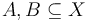$A,B \subseteq X$ are disjoint closed subsets, there exist open sets$C,D$ of$X$ containing$A$ and$B$ respectively such that$C$ and$D$ are disjoint.

Proof:

1. For every$a \in A$, there exist open sets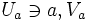$U_a \ni a, V_a$ containing$B$, such that$U_a$ and$V_a$ are disjoint. This follows from regularity.
2. The$U_a$s form a collection of open subsets of$X$ covering$A$. Along with$X \setminus A$, these form an open cover of$X$. This has a locally finite open refinement. Throwing out from this any open subset not intersecting$A$, we still get a locally finite collection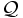$\mathcal{Q}$ of open subsets, each contained in some$U_a$, that cover$A$. Let$C$ be the union of all members of$\mathcal{Q}$.
3. For any$b \in B$, there exists an open subset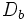$D_b$ around$b$ that does not intersect$C$: First, there exists an open subset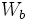$W_b$ around$b$ intersecting only finitely many members of$\mathcal{Q}$. Let$T$ be a finite subset of$A$ that contains, for each of this finite list of members of$\mathcal{Q}$, a point$a$ such that that member is contained in$U_a$. Then,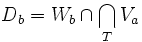$D_b = W_b \cap \bigcap_T V_a$ works.
4. Let$D$ be the union of all$D_b$s,$b \in B$. Then,$C$ and$D$ are the required disjoint open subsets: This follows from the previous step.